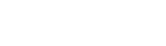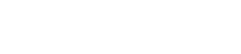# Gas offtake flow calculator

## calculation of gas offtake from the pipe or through the valve

### online since 2012## Calculator start selection

Read all about available deployments. In any way of utilizing calculator, Internet connection is not required, but nice to have for authentication at least.

save/open multiple results
export to word and excel
print results
custom fluid properties
K factor for fittings, resistance coefficient
pipe surface roughness selection
select between gauge and absolute pressure
compressible isothermal flow
dry air isothermal flow
gas offtake flow
natural gas flow

Administrator role not needed to install calculator

#### When is this calculator suitable?

Calculation of gas offtake from the pipe or through the valve. You can use the calculator for flow rate or pipe diameter calculation when the gas is exiting from a pipe or a reservoir to the atmosphere, or to some other pipe or a reservoir.

You can use the calculator for flow in pipelines that include valves and fittings. You can calculate the maximum flow rate when you know pressure difference and pipe diameter, or you can calculate pipe diameter when you know flow rate and pressure drop.

The calculator is applicable for all two and three atomic gases, like air, nitrogen, carbon dioxide and other gases. The calculator is suitable for steady flow with constant pressures at one point of streamline.

#### What are the calculator restrictions?

The calculator is suitable for ideal gases, as the calculator uses the equation of state for ideal gas during calculation. The calculator is not applicable for non-steady, pulsating flow.

#### How is the calculation executed?

Based on the known pressure difference (head loss) between one point of flow stream at the start of a pipe or in front of a valve, to the outside point (like atmosphere), or after a valve with known inside pipe diameter, mass flow rate, and volume flow rate is calculated. The calculator uses a modified Darcy formula for flow rate calculation.where is:

w - offtake mass flow rate [kg/s]
Y - expansion factor [ - ]
d - internal pipe diameter [mm]
Δp - pressure drop [Pa]
ρ - density [kg/m3]
K - resistance coefficient [ - ]

The calculator is calculating a friction coefficient using the Colebrook-White formula:where is:

f - friction factor [ - ]
k - pipe roughness [mm]
D - internal pipe diameter [mm]
Re - Reynolds number [ - ]

The calculator presents Reynolds number and expansion factor as calculation results.

The calculator is checking if a flow is choked or not and presents choked flow status. For choked flow conditions, the calculator is calculating the flow rate for that condition.

#### What else has to be known to perform the calculation?

You should enter resistance factor K for valves and fittings if they exist in the pipeline as well as pipeline surface roughness.

#### When is this calculator not relevant?

The calculator is not relevant for liquids.

# Need a helping hand?If you need a quick calculation, but you are not still familiar how to use the calculator, you can order calculation service from the calculator developer.